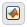## Operations for Complex Data in Stateflow

Stateflow® charts in Simulink® models have an action language property that defines the syntax that you use to compute with complex data. The action language properties are:

•MATLAB® as the action language.

•C as the action language.

For more information, see Differences Between MATLAB and C as Action Language Syntax.

### Notation for Complex Data

In charts that use MATLAB as the action language, you can define complex data by using complex number notation `a + bi`, where `a` and `b` are real numbers. For example, this statement assigns a value of 3+4i to `x`:

`x = 3 + 4i;`

Alternatively, you can define complex data by using the `complex` operator:

```complex(<real_part>,<imag_part>) ```

`<real_part>` and `<imag_part>` are arguments that define the real and imaginary parts of the complex number, respectively. The two arguments must be real values or expressions that evaluate to real values. As in the preceding example, this statement assigns a value of 3+4i to `x`:

`x = complex(3,4);`

Charts that use C as the action language do not support complex number notation `a + bi`. To define a complex number based on two real values, use the `complex` operator.

### Binary Operations

This table summarizes the interpretation of all binary operations on complex operands according to their order of precedence (1 = highest, 3 = lowest). Binary operations are left associative so that, in any expression, operators with the same precedence are evaluated from left to right.

Operation

Precedence

MATLAB as the Action Language

C as the Action Language

`a * b`

1

Multiplication.

Multiplication.

`a / b`

1

Division.

Not supported. Use the `\` operation in a MATLAB function. See Perform Complex Division with a MATLAB Function.

`a + b`

2

`a - b`

2

Subtraction.

Subtraction.

`a == b`

3

Comparison, equal to.

Comparison, equal to.

`a ~= b`

3

Comparison, not equal to.

Comparison, not equal to.

`a != b`

3

Not supported. Use the operation ```a ~= b```.

Comparison, not equal to.

`a <> b`

3

Not supported. Use the operation ```a ~= b```.

Comparison, not equal to.

### Unary Operations and Actions

This table summarizes the interpretation of all unary operations and actions on complex data. Unary operations:

• Have higher precedence than the binary operators.

• Are right associative so that, in any expression, they are evaluated from right to left.

Operation

MATLAB as the Action Language

C as the Action Language

`-a`

Negative.

Negative.

`a++`

Not supported. Use the expression ```a = a+1```.

Increment. Equivalent to ```a = a+1```.

`a--`

Not supported. Use the expression ```a = a-1```.

Decrement. Equivalent to ```a = a-1```.

### Assignment Operations

This table summarizes the interpretation of assignment operations in Stateflow charts.

Operation

MATLAB as the Action Language

C as the Action Language

`a = b`

Simple assignment.

Simple assignment.

`a += b`

Not supported. Use the expression ```a = a+b```.

Equivalent to ```a = a+b```.

`a -= b`

Not supported. Use the expression ```a = a-b```.

Equivalent to ```a = a-b```.

`a *= b`

Not supported. Use the expression ```a = a*b```.

Equivalent to ```a = a*b```.

### Access Real and Imaginary Parts of a Complex Number

To access the real and imaginary parts of a complex number, use the `real` and `imag` operators.

#### `real` Operator

The `real` operator returns the value of the real part of a complex number:

`real(<complex_expr>)`

`<complex_expr>` is an expression that evaluates to a complex number. For example, if `frame(200)` evaluates to the complex number `8.23 + 4.56i`, this expression returns a value of `8.2300`:

`real(frame(200))`

#### `imag` Operator

The `imag` operator returns the value of the imaginary part of a complex number:

`imag(<complex_expr>)`

`<complex_expr>` is an expression that evaluates to a complex number. For example, if `frame(200)` evaluates to the complex number `8.23 + 4.56i`, this expression returns a value of `4.5600`:

`imag(frame(200))`